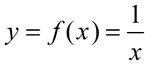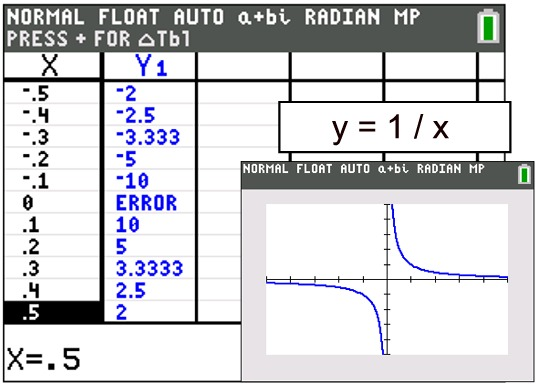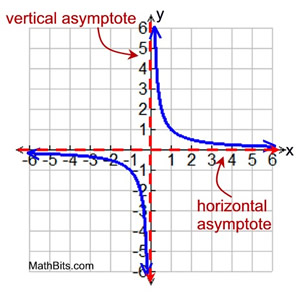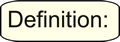Graphing Rational Functions MathBitsNotebook.com Terms of Use   Contact Person: Donna RobertsLet's start by examining the graph of a simple rational function. You may have seen this graph in the past. Due to the restrictions that are placed on the x-values of rational functions, you can guess that their graphs are going to be more complicated to predict around these undefined locations.;     x ≠ 0

 Notice, in the table, the behavior of the graph around its undefined value at x = 0. The function changes its sign. Its values are negative to the left of 0, but they are positive to the right of 0.Also, notice what is happening to the graph surrounding x = 0.
• As the graph approaches x = 0 from the right, its y-values are getting increasingly larger.
As x → 0+, y → + ∞.
( 0+ approaches from right, 0- approaches from left)

• As the graph approaches x = 0 from the left, its y-values are getting increasingly smaller.
As x → 0-, y → - ∞.

• x = 0 is a vertical asymptote for this graph.A vertical asymptote is a vertical straight line toward which a function approaches closer and closer, but never reaches (or touches). Vertical asymptotes correspond to the undefined locations of rational functions.

Also, notice how the graph is "approaching" the x-axes at the far right and far left.
• As x gets increasingly larger, (x → ∞), the y-values are positive and are getting closer and closer to 0 (the x-axis), but they will never actually get to zero. They will just continue to get closer and closer to zero (the x-axis).

• As x gets increasingly smaller, (x → - ∞), the y-values are negative and are also getting closer and closer to 0 (the x-axis), but they will never actually get to zero. They will also just continue to get closer and closer to zero.

y = 0 is a horizontal asymptote for this graph.

 The behavior of the graph in relation to a vertical asymptote is clear cut and constant: the graph NEVER touches them. Never! The behavior of the graph in relation to a horizontal asymptote, however, is not as clearly defined. You may only approach a horizontal asymptote, or you may touch a horizontal asymptote, or you may even cross a horizontal asymptote. Horizontal asymptotes give more of a general impression of what the graph is doing, and are generally associated with the far ends of the graph.Don't Panic!!! In Algebra 2, you only need to have a general understanding of this type of graph so you will know what you are seeing on your graphing calculator, and how the graph relates to the restrictions on a rational function.How to sketch a rational function without a graphing calculator:
(and yes, this will be a "rough", but sufficient, sketch)

 1. As with all functions, find the intercepts: • for the x-intercept set y = 0 • for the y-intercept set x = 0 Beware: As we saw in the graph above, rational functions may not have any intercepts. 2. Find the vertical asymptote(s): • set the denominator = 0 and solve 3. Find the horizontal asymptote(s): (assuming the rational function is expressed as a single fraction) • get the degree of the numerator, n, such as axn • get the degree of the denominator, m, such as bxm • if n < m, the x-axis is the horizontal asymptote • if n = m, the line y = a/b is the horizontal asymptote • if n > m, there is no horizontal asymptote 4. Use the vertical asymptote(s) to divide the x-axis into regions. • Graph at least one point in each region. • Use this point to determine the position of the graph in relation to the horizontal asymptote(s). • It may be necessary to graph additional points to get the shape of the graph. 5. Sketch the graph.